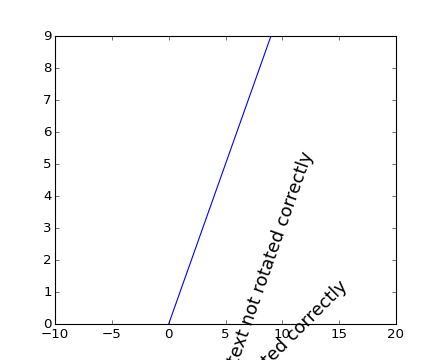### We're updating the default styles for Matplotlib 2.0

Learn what to expect in the new updates#### Previous topic

pylab_examples example code: text_rotation.py

#### Next topic

pylab_examples example code: titles_demo.py

# pylab_examples example code: text_rotation_relative_to_line.py¶```#!/usr/bin/env python
"""
Text objects in matplotlib are normally rotated with respect to the
screen coordinate system (i.e., 45 degrees rotation plots text along a
line that is in between horizontal and vertical no matter how the axes
are changed).  However, at times one wants to rotate text with respect
to something on the plot.  In this case, the correct angle won't be
the angle of that object in the plot coordinate system, but the angle
that that object APPEARS in the screen coordinate system.  This angle
is found by transforming the angle from the plot to the screen
coordinate system, as shown in the example below.
"""
from pylab import *

# Plot diagonal line (45 degrees)
h = plot( r_[:10], r_[:10] )

# set limits so that it no longer looks on screen to be 45 degrees
xlim([-10,20])

# Locations to plot text
l1 = array((1,1))
l2 = array((5,5))

# Rotate angle
angle = 45
trans_angle = gca().transData.transform_angles(array((45,)),
l2.reshape((1,2)))

# Plot text
th1 = text(l1,l1,'text not rotated correctly',fontsize=16,
rotation=angle)
th2 = text(l2,l2,'text not rotated correctly',fontsize=16,
rotation=trans_angle)

show()
```

Keywords: python, matplotlib, pylab, example, codex (see Search examples)